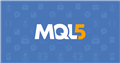# Margin

Hello! I have one question:

If I calculate margin like this:

```   double lot=0;
for(int i =OrdersTotal()-1; i>=0; i--)
{
if(OrderSelect(i,SELECT_BY_POS))
{

lot+= OrderLots();

}
}

int leverage= (int)AccountInfoInteger(ACCOUNT_LEVERAGE);
double marginterminal=AccountInfoDouble(ACCOUNT_MARGIN);

double mmargin= lot*100000/leverage;

Print("marginterminal=",marginterminal);
Print("calcmargin=",mmargin); ```

it is a little different then this

`AccountInfoDouble(ACCOUNT_MARGIN)`

The results are like this:

2022.04.11 19:18:35.732 test EURUSD,M4: marginterminal=298.9966666666667

2022.04.11 19:18:35.732 test EURUSD,M4: calcmargin=289.9999999999999

Why?Documentation on MQL5: Constants, Enumerations and Structures / Environment State / Symbol Properties
• www.mql5.com
Symbol Properties - Environment State - Constants, Enumerations and Structures - MQL5 Reference - Reference on algorithmic/automated trading language for MetaTrader 5

I am not sure about your difference, but you have hard coded the contract size, are not taking into account quotes between symbol currencies and account currencies.

I would guess, the terminal function does that and in this case your calculations and the terminal calculations differ by one more operation, introducing the difference you are seeing.

Dominik Christian Egert #:
I am not sure about your difference, but you have hard coded the contract size, are not taking into account quotes between symbol currencies and account currencies.

I would guess, the terminal function does that and in this case your calculations and the terminal calculations differ by one more operation, introducing the difference you are seeing.

Thank you ... I dont understand what do you mean with contract size...I use 100000 which is for FX pairs.... this is just for test...if I have used MarketInfo(_Symbol,MODE_LOTSIZE) the result would be the same